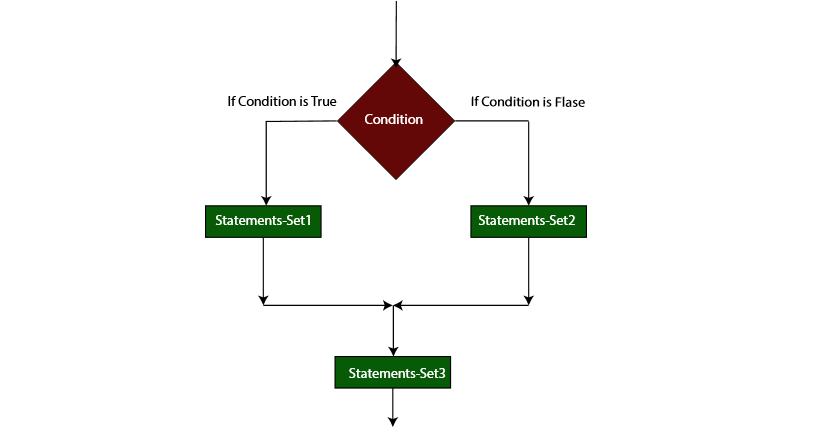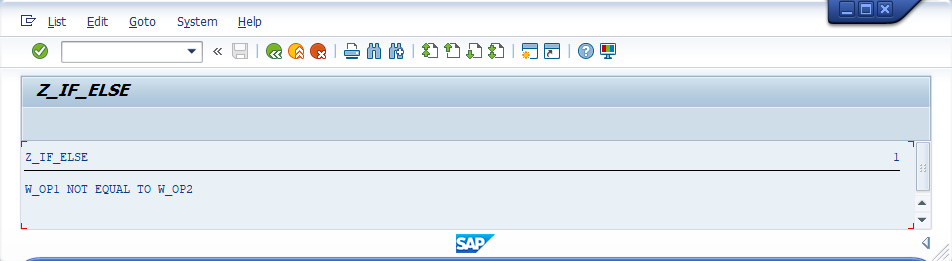###### Summary -

In this topic, we described about the below sections -

IF statement consists of a one or more logical expressions/Conditions followed by one or more statements. IF statement followed by ELSE contains another set of statements.

The set of statements in IF statement gets executed when the logical expression/condition is true. The set of statements with ELSE part gets executed when the logical expression/condition is false.

ENDIF scope terminator is mandatory with IF..ELSE statement. Each statement in IF..ELSE statement should ends with period(.) including IF, ELSE and ENDIF.

#### Syntax -

``````IF <logical-expression>.
.
Statements-set1.
.
ELSE.
.
Statements-set2.
.
ENDIF.
Statement-set3.
``````

Below diagram describes the flow of IF ELSE statement -If the specified logical-expression is determined as true, then the statements-set1 executed. Next, the control transfers to statements-set3. If the specified logical-expression is determined as false, then the statements-set2 executed. Next, the control transfers to statements-set3.

#### Example -

Below example shows how the IF ELSE statement coded in the application program.

#### Code -

``````*&---------------------------------------------------------------------*
*& Report  Z_IF_ELSE
*&---------------------------------------------------------------------*
*& Written by TutorialsCampus
*&---------------------------------------------------------------------*

REPORT  Z_IF_ELSE.

* Declaring Variable
DATA: W_OP1 TYPE I VALUE 90,
W_OP2 TYPE I VALUE 70.

* IF condition to verify both variables are equal or not
IF W_OP1 EQ W_OP2.

* If both variables are equal, displays below message.
WRITE 'W_OP1 EQUAL TO W_OP2'.
ELSE.

* If both variables are not equal, displays below message.
WRITE 'W_OP1 NOT EQUAL TO W_OP2'.
ENDIF.
``````

#### Output -#### Explaining Example -

In the above example, each and every statement is preceeded with a comment to explain about the statement. Go through them to get clear understanding of example code.

IF W_OP1 EQ W_OP2, verifies W_OP1 and W_OP2 are equal or not. If equal, displays 'W_OP1 EQUAL TO W_OP2'. otherwise, 'W_OP1 NOT EQUAL TO W_OP2' gets displayed.